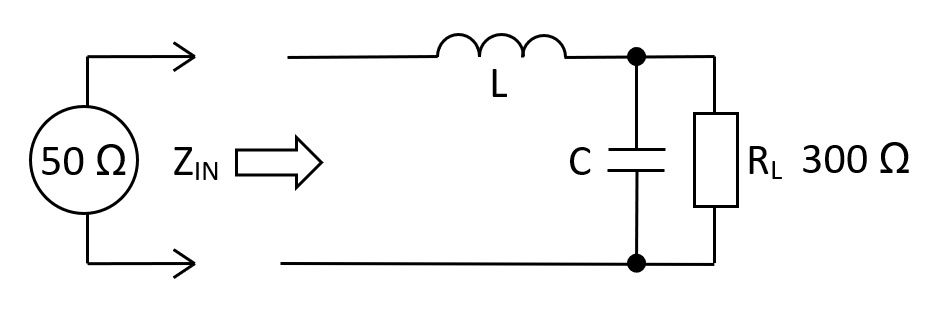At 10 MHz, a 50 Ω source driving a 300 Ω load via a low-pass L-pad transformer requires a capacitance of 118.62 pF and an inductance of 1.779 μH. Previously it was shown that using the lossy resistive impedance transformer, the attenuation would be 5.63 dB into a 300 Ω.

$$\hspace{18.9cm}$$

Relevant formulas$$\boxed{L = R_{IN}\cdot R_L\cdot C}\hspace{0.3cm}\text{and}\hspace{0.3cm}\boxed{C = \dfrac{1}{\omega R_L}\sqrt{\frac{R_L}{R_{IN}}-1}}$$

 RIN: Ω RL: Ω FIN: MHz L: µH C: pF Fn:      Natural resonant frequency of the circuit MHz AV at FIN: Voltage gain from $$Z_{IN}$$ to $$R_L$$ V/V

Values for L and C

The formulas are found by examining $$Z_{IN}$$ and finding the frequency ($$\omega_r$$) where it's resistive $$(R_{IN})$$. We then move on to calculate $$L$$ in terms of $$R_{IN}, C$$ and $$R_L$$. The input impedance $$Z_{IN}$$ is: -

$$\hspace{1.3cm}Z_{IN} = j\omega L + \dfrac{\dfrac{R_L}{j\omega C}}{R_L + \dfrac{1}{j\omega C}} = j\omega L +\dfrac{R_L}{1 + j\omega R_L C} = j\omega L +\dfrac{R_L}{1+\omega^2R_L^2C^2} - \dfrac{j\omega R_L^2C}{1+\omega^2R_L^2C^2}$$

With imaginary terms at zero we can say: $$R_{IN} = \dfrac{R_L}{1+\omega_r^2R_L^2C^2}$$. This gives us the value for $$C$$: - $$\color{green}{\boxed{C = \dfrac{1}{\omega_rR_L}\cdot\sqrt{\dfrac{R_L}{R_{IN}}-1}}}$$ And we can equate imaginary terms to zero to locate $$L$$: - $$\color{red}{\boxed{L = \dfrac{R_L^2C}{1+\omega_r^2R_L^2C^2}}}$$ We can use $$\omega_r^2$$ from the green boxed $$C$$ formula to further reduce $$L$$: - $$\color{red}{\boxed{L =\dfrac{R_L^2C}{1 + \dfrac{R_L}{R_{IN}}-1}=R_{IN}R_LC}}$$

Gain at the operating frequency

To calculate the gain at $$F_{IN}$$, use the 2nd order low-pass filter transfer formula. Recognize that $$\omega_n$$ (natural resonant frequency) and $$\zeta$$ (parallel damping ratio) formulas are involved: -

$$\hspace{0.5cm}|H(j\omega)| = \dfrac{1}{\sqrt{1 +\dfrac{\omega^2}{\omega_n^2}\cdot(4\zeta^2-2) +\dfrac{\omega^4}{\omega_n^4}}}$$ $$\hspace{0.25cm}$$ where $$\omega_n^2 = \dfrac{1}{LC}$$ and, $$\hspace{0.25cm}\zeta = \dfrac{1}{2R_L}\cdot\sqrt{\dfrac{L}{C}}\hspace{0.5cm}$$

Because we know this from earlier: $$\hspace{0.5cm}\color{red}{\omega = \sqrt{\dfrac{1}{LC} - \dfrac{1}{R_L^2 C^2}}}\hspace{0.5cm}$$ we can say this: -

$$\dfrac{\omega^2}{\omega_n^2} = \left(\dfrac{1}{LC} - \dfrac{1}{R_L^2 C^2}\right)\cdot LC\hspace{0.25cm}= \hspace{0.25cm}1- \dfrac{L}{R_L^2C}$$

From above $$\dfrac{L}{R_L^2C} = 4\zeta^2$$ hence: -

$$|H(j\omega)| = \dfrac{1}{\sqrt{1 +(1-4\zeta^2)\cdot(4\zeta^2-2) +(1-4\zeta^2)^2}}$$

$$|H(j\omega)| = \dfrac{1}{\sqrt{1 +(4\zeta^2 - 2 - 16\zeta^4 + 8\zeta^2) +(1-8\zeta^2+16\zeta^4)}}$$

$$|H(j\omega)| = \dfrac{1}{\sqrt{4\zeta^2}} = \dfrac{1}{2\zeta} = Q$$

This is an interesting result; the gain at $$F_{IN}$$ equals the quality factor of the $$R_LLC$$ circuit. The only other point that this happens is at $$F_n$$ but then, the input impedance isn't resistive.

Apart from the losses in the source's series output resistance, the power transferred is 100%. Using the default example of 50 Ω and 300 Ω, the voltage gain is 2.44949 so, if the source voltage is 1 volt, the actual input voltage to the inductor will be 0.5 volts at 10.000 MHz.

This means the voltage across the 300 Ω load is 1.224745 volts. This is a power of 5 mW. The power into the inductor is 0.5 volts into 50 Ω and that is also a power of 5 mW.